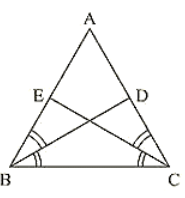×#### Thank you for registering.

One of our academic counsellors will contact you within 1 working day.

Click to Chat

1800-1023-196

+91-120-4616500

CART 0

• 0

MY CART (5)

Use Coupon: CART20 and get 20% off on all online Study Material

ITEM
DETAILS
MRP
DISCOUNT
FINAL PRICE
Total Price: Rs.

There are no items in this cart.
Continue Shopping• Complete JEE Main/Advanced Course and Test Series
• OFFERED PRICE: Rs. 15,900
• View Details

```Chapter 10: Congruent Triangles Exercise – 10.2

Question: 1

Given that RT = TS, ∠1 = 2, ∠2 and 4 = 2 ∠(3) Prove that ΔRBT  ≅ ΔSAT.

Solution:

In the figure, given that

RT = TS   .... (i)

∠1 = 2∠2  .... (ii)

And ∠4 = 2∠3  .... (iii)

To prove that ΔRBT ≅ ΔSAT.

Let the point of intersection RB and SA be denoted by O

Since RB and SA intersect at O

∠AOR = ∠BOS          [Vertically opposite angles]

∠1 = ∠4

2∠2 = 2∠3 [From (ii) and (iii)]

∠2 = ∠3  ….(iv)

Now we have RT = TS in ΔTRS

ΔTRS is an isosceles triangle

∠TRS = ∠TSR .... (v)

But we have

∠TRS = ∠TRB + ∠2  .....(vi)

∠TSR = ∠TSA + ∠3  .... (vii)

Putting (vi) and (vii) in (v) we get

∠TRB + ∠2 =  ∠TSA + ∠B

⟹ ∠TRB = ∠TSA        [From (iv)]

Now consider ΔRBT and ΔSAT

RT = ST [From (i)]

∠TRB = ∠TSA            [From (iv)] ∠RTB = ∠STA    [Common angle]

From ASA criterion of congruence, we have

ΔRBT = ΔSAT

Question: 2

Two lines AB and CD intersect at O such that BC is equal and parallel to AD. Prove that the lines AB and CD bisect at O.

Solution:

Given that lines AB and CD Intersect at O.We have to prove that AB and CD bisect at O.

To prove this first we have to prove that ΔAOD ≅ ΔBOC

Question: 2

BD and CE are bisectors of ∠B and ∠C of an isosceles ΔABC with AB = AC. Prove that BD = CE

Solution:

Given that ΔABC is isosceles with AB = AC and BD and CE are bisectors of ∠B and ∠ C We have to prove BD = CE

Since AB = AC

⟹ ΔABC = ΔACB ... (i)

[Angles opposite to equal sides are equal]

Since BD and CE are bisectors of ∠B and ∠C

∠ABD = ∠DBC = ∠BCE = ECA = ∠B/2 = ∠C/2

Now,Consider ΔEBC = ΔDCB

∠EBC = ∠DCB [∠B = ∠C] [From (i)]

BC = BC [Common side]

∠BCE = ∠CBD [From (ii)]

So, by ASA congruence criterion, we have Δ EBC ≅ Δ DCB

Now,

CE = BD [Corresponding parts of congruent triangles we equal]

or, BD = CE

Hence proved

Since AD ∥ BC and transversal AB cuts at A and B respectively

∠DAO = ∠OBC .... (ii) [alternate angle]

And similarly AD ∥ BC and transversal DC cuts at D and C respectively

∠ADO = ∠OBC   …. (iii) [alternate angle]

Since AB end CD intersect at O.

∠AOD = ∠BOC    [Vertically opposite angles]

Now consider ΔAOD and ΔBOD

∠DAO = ∠OBC [From (ii)]

And ∠ADO = ∠OCB  [From (iii)]

So, by ASA congruence criterion, we have

ΔAOD ≅ ΔBOC

Now,

AO = OB and DO = OC [Corresponding parts of congruent triangles are equal)

Lines AB and CD bisect at O.

Hence proved
```### Course Features

• 728 Video Lectures
• Revision Notes
• Previous Year Papers
• Mind Map
• Study Planner
• NCERT Solutions
• Discussion Forum
• Test paper with Video Solution